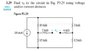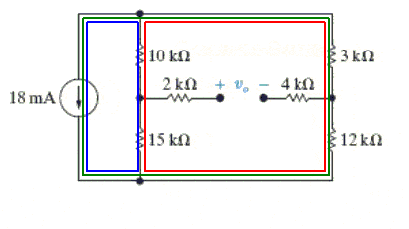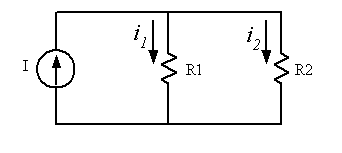# Voltage/Current Division with central Voltage Source

• Bluestribute
That is not what I wrote.In summary, the conversation involved finding V0 using voltage/current division and the equations V=(VsR)/Req and I=(IsIeq)/I. The person used general circuit rules to calculate the total resistance but was unsure of what to do next. They were reminded to include units in their calculations and to use current and voltage division to find the branch currents and potential drops across the individual resistors in those branches. They were also given hints on how to identify and choose the appropriate loops for their circuit.

## Homework Statement

Find V0 using voltage/current division

V=(VsR)/Req
I=(IsIeq)/I

## The Attempt at a Solution

I used general circuit rules (parallel and series) to get the total resistance as 14.775 ohms{?}. And I'm stuck with what to do next . . .

#### Attachments

•ks.jpg
10.7 KB · Views: 763
Show your work. How did you calculate "total resistance"?

Ah yes. I was going to do that but was in a hurry.

[(10+3)//(15+12)] + 4 + 2 = 14.775

That's part one that's tripping me up. Part two is using the those division equations to find the source voltage . . .

So, by "total resistance" you mean the Thevenin resistance as seen at the open terminals. Okay. Be sure to include units on all results. All the resistors in your circuit have units of kΩ, so what should be the units of your result by the method you've used?

As for the current division, what are the resistances of the two branches that the 18 mA sees?

So yes, I'm in K-Ohms. The 18mA sees a 13 K-Ohm and 27 K-Ohm branch, correct? We haven't quite got to Thevenin in this class, or just ignored it maybe, but sure. From the vague recollection I have of it from last semester, learning from these forums.

Bluestribute said:
So yes, I'm in K-Ohms.
Right.
The 18mA sees a 13 K-Ohm and 27 K-Ohm branch, correct?
Nope. Trace the paths that the current can flow from the current source, around a loop, and back to the current source. Remember that the Vo terminals are open: no current can flow across an open path.
We haven't quite got to Thevenin in this class, or just ignored it maybe, but sure. From the vague recollection I have of it from last semester, learning from these forums.
Well, you don't really need Thevenin or Norton here (although they could be used). Which is why I was curious about your total resistance calculation; I didn't see how it was going to be useful if you're just looking to find Vo. Current division and voltage division is the way to go here.

So it would be going through +15 and +10 K-Ohm resistors (they + is just saying it's going through the positive terminal to negative . . . )? So do that with all 3 loops (well, I guess meshes in this case)?

And is it legal to substitute V0R in for "i" across the resistors? I'm just remembering my notes and we did something similar though the context has been lost. But anyways, do 3 loops?

Bluestribute said:
So it would be going through +15 and +10 K-Ohm resistors (they + is just saying it's going through the positive terminal to negative . . . )? So do that with all 3 loops (well, I guess meshes in this case)?
You don't need any loop equations if you're going to use current and voltage division. You just need to identify the two branches in parallel with the current source that the current will flow through. You've identified one so far (the 10 k +15 k branch).
And is it legal to substitute V0R in for "i" across the resistors? I'm just remembering my notes and we did something similar though the context has been lost. But anyways, do 3 loops?
No, there's no current associated with Vo as it's an open circuit.

Just identify the two branches that parallel the current source and use current division o determine the current in each of them. Then you can play with voltage division to find the potential on either side of the open terminals, hence Vo.

You say 2 loops but I see 3? Why would you ignore the 2k and 4k Ohm resistors on the inside (I'm assuming the second loop doesn't include them)? Or does it not matter which loop I choose for number 2?

Bluestribute said:
You say 2 loops but I see 3? Why would you ignore the 2k and 4k Ohm resistors on the inside (I'm assuming the second loop doesn't include them)? Or does it not matter which loop I choose for number 2?
Vo is an open circuit. There is no path for current there, hence no loop current, hence not a loop. You cannot make a loop that includes an open circuit.

Here are the three possible loops for your circuit. Any two of them will produce linearly independent equations, the third will produce redundant information. Hint: You only every need enough loops so that each current-carrying component in the circuit is included in the path of at least one loop.

Here are the three possible loops for your circuit:So is the first loop equation essentially 18mA + V15/15K + V10/10K = 0?

And then doing the entire loop gives 18mA + V12/15K + V3/10K = 0?

Bluestribute said:
So is the first loop equation essentially 18mA + V15/15K + V10/10K = 0?

And then doing the entire loop gives 18mA + V12/15K + V3/10K = 0?
You seem to be introducing a lot of unknowns and setting yourself up for a lot of extra work.

Why not just use current division to find the branch currents, then voltage division to finds the potentials at the resistor junctions? Very straight forward and you have all the information you require.

So 18mA + i15 + i10 = 0? And same with the entire loop. Then how do I find voltage with that? Don't you need a "V" in there somewhere?

Okay, current division.

Consider the following circuit:The current from the source, ##I##, will be divided into the two parallel branches as ##i_1## and ##i_2##. The branches have total resistances R1 and R2 respectively. One can determine the values of those currents from the total current ##I## and the branch resistances. Namely:
$$i_1 = I \frac{R2}{R1 + R2}$$
$$i_2 = I \frac{R1}{R1 + R2}$$
So you're in a position to determine the currents in the branches of your circuit. With those currents you can determine the potential drops across the individual resistors in those branches...

So i1 = 18mA(25KΩ/40KΩ) and i2 = 18mA(15KΩ/40KΩ)?

Bluestribute said:
So i1 = 18mA(25KΩ/40KΩ) and i2 = 18mA(15KΩ/40KΩ)?
No, you didn't pay attention to which resistance forms the numerator of the ratio. Revisit post #14 and look closely at the expressions.

I ended up just getting a solutions manual since I realized it'll be a long semester . . . and unless I make it click my way, it never will.

So it solved each branch first. I understand that, using the parallel knowledge (and that's circuit division, yes?). IE [(10+15)//(3+12)]/(10+15) * 18

The left most was 6.75 mA, and the right most 11.25 mA. The numerators were Req, and the denominators were just the branch resistances in series.

Then they calculated voltage across each resistor towards the top, which I also understand. IE V15 = -(6.75)(15) because of the direction of the current source.

The final equation they got was V = -101.25- -135. I understand everything up until the end . . . Wouldn't that entire "lower wire" (is node appropriate?) be one voltage? Why the subtraction?

Bluestribute said:
I ended up just getting a solutions manual since I realized it'll be a long semester . . . and unless I make it click my way, it never will.

So it solved each branch first. I understand that, using the parallel knowledge (and that's circuit division, yes?). IE [(10+15)//(3+12)]/(10+15) * 18

The left most was 6.75 mA, and the right most 11.25 mA. The numerators were Req, and the denominators were just the branch resistances in series.
No, the numerators were the resistances of the other branch, the denominators were the total resistance of both branches. The current divider is an important concept, so it'll crop up in exams. Be sure to remember the procedure: The current in one branch is equal to the total current multiplied by the ratio of the resistance of the other branch to the total resistance of both branches.
Then they calculated voltage across each resistor towards the top, which I also understand. IE V15 = -(6.75)(15) because of the direction of the current source.

The final equation they got was V = -101.25- -135. I understand everything up until the end . . . Wouldn't that entire "lower wire" (is node appropriate?) be one voltage? Why the subtraction?
The calculation is for the potential across the terminals where Vo is indicated. According to the figure, Vo is the potential at the left terminal minus the potential at the right terminal (the diagram puts the + of the voltage is on the left, the - on the right). So they calculated the potential at each terminal with respect to some reference node (the bottom node) and then calculated the difference in the potentials at the terminals.

The potentials at the terminals are the same as the potentials at the junctions of the resistors of the branches (since no current can flow through the 2 K or 4 K resistors). Choosing a common reference node (the bottom rail), they found the potential drops across the 15k and 12k resistors using the branch currents, and thus determined the potentials at those junctions with respect to that reference node.

But the numerators had the parallel notation?

I understand the last part now. So using general knowledge of the equal voltages across parallel branches, where the "top" and "bottoms" are the same voltage. But now that first part is confusing me since apparently they did it wrong? How did they get parallel notation in the numerator?

Bluestribute said:
But the numerators had the parallel notation?

I understand the last part now. So using general knowledge of the equal voltages across parallel branches, where the "top" and "bottoms" are the same voltage. But now that first part is confusing me since apparently they did it wrong? How did they get parallel notation in the numerator?
Sorry, I don't follow. Perhaps I haven't directly seen their work.

There are two parallel branches supplied by a current source. Current division using the branch resistances give you the branch currents. Each branch is also a voltage divider, since there are two resistors in each branch. Using the branch current and the resistance values the potential difference at the junction of the resistors can be determined with respect to a reference node (choose the top or bottom rail as the reference and the resistor between the reference node and the junction as the device across which the potential with respect to the reference node is to be determined). Once the potentials at the junctions are established you can determine the potential difference between the junctions.

Bluestribute said:
Then they calculated voltage across each resistor towards the top, which I also understand. IE V15 = -(6.75)(15) because of the direction of the current source.

The final equation they got was V = -101.25- -135.
You need to calculate the voltage at the junction of the 10k and 15k resistors. You also need to calculate the voltage at the junction of the 3k and 12k resistors. Then you subtract these two voltages, and there's your answer.

What I inferred (and it worked), was that each branch used current division to find the current through the branch. Then, using the branch currents, the "top and bottom" voltages were found (because V0 will be the starting point at each "upper" node of the branch)